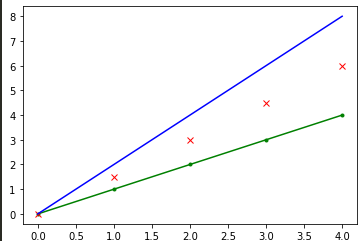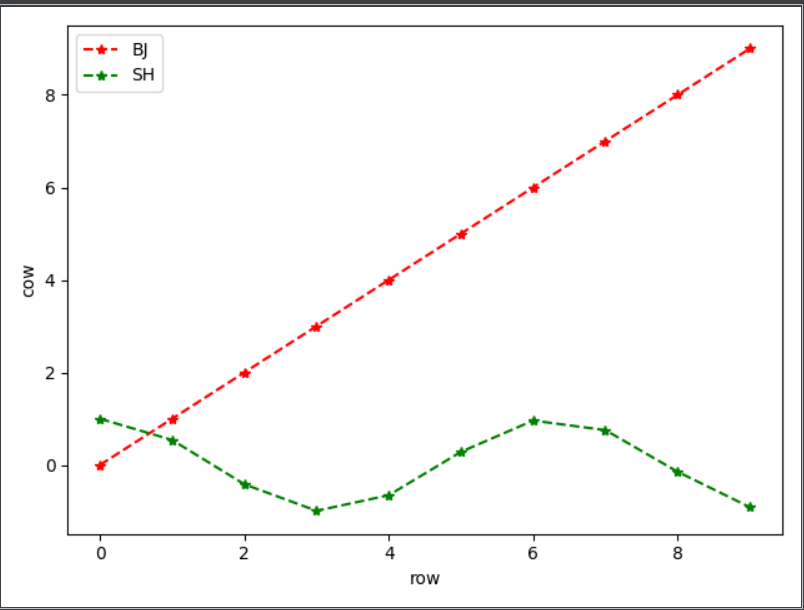# plt.plot()函数

``````plt.plot(x, y, format_string, **kwargs)
``````

`x` X轴数据，列表或数组，可选
`y` Y轴数据，列表或数组
`format_string` 控制曲线的格式字符串，可选
`**kwargs` 第二组或更多(x,y,format_string)，可画多条曲线

`format_string` 由颜色字符、风格字符、标记字符组成

• 颜色字符
• `'b'` 蓝色 `'m'` 洋红色 magenta
• `'g'` 绿色 `'y'` 黄色
• `'r'` 红色 `'k'` 黑色
• `'w'` 白色 `'c'` 青绿色 cyan
• `'#008000'` RGB某颜色 `'0.8'` 灰度值字符串
• 多条曲线不指定颜色时，会自动选择不同颜色
• 风格字符
• `'‐'` 实线
• `'‐‐'` 破折线
• `'‐.'` 点划线
• `':'` 虚线
• `'' ' '` 无线条
• 标记字符
• `'.'` 点标记
• `','` 像素标记(极小点)
• `'o'` 实心圈标记
• `'v'` 倒三角标记
• `'^'` 上三角标记
• `'>'` 右三角标记
• `'<'` 左三角标记…等等
• `**kwargs` : 第二组或更多(x,y,format_string)

`color` : 控制颜色, color=‘green’
`linestyle` : 线条风格, linestyle=‘dashed’
`marker` : 标记风格, marker=‘o’
`markerfacecolor`: 标记颜色, markerfacecolor=‘blue’
`markersize`: 标记尺寸, markersize=20

``````b = np.arange(5)
plt.plot(b,b*1.0,'g.-',b,b*1.5,'rx',b,b*2.0, 'b')
plt.show()
``````# plt.scatter()函数

plt.scatter()函数用于生成一个scatter散点图。

``````matplotlib.pyplot.scatter(x, y, s=20, c='b', marker='o', cmap=None, norm=None, vmin=None, vmax=None, alpha=None, linewidths=None, verts=None, hold=None, **kwargs
``````
参数 解释说明
x，y 表示的是shape大小为(n,)的数组，也就是我们即将绘制散点图的数据点，输入数据。
s 表示的是大小，是一个标量或者是一个shape大小为(n,)的数组，可选，默认20。
c 表示的是色彩或颜色序列，可选，默认蓝色’b’。但是c不应该是一个单一的RGB数字，也不应该是一个RGBA的序列，因为不便区分。c可以是一个RGB或RGBA二维行数组。
marker MarkerStyle，表示的是标记的样式，可选，默认’o’。
cmap Colormap，标量或者是一个colormap的名字，cmap仅仅当c是一个浮点数数组的时候才使用。如果没有申明就是image.cmap，可选，默认None。
norm Normalize，数据亮度在0-1之间，也是只有c是一个浮点数的数组的时候才使用。如果没有申明，就是默认None。
vmin，vmax 标量，当norm存在的时候忽略。用来进行亮度数据的归一化，可选，默认None。
alpha 标量，0-1之间，可选，默认None。
linewidths 标记点的长度，默认None。

例子

``````import numpy as np
import matplotlib.pyplot as plt

np.random.seed(0)
x=np.random.rand(20)
y=np.random.rand(20)

area=(50*np.random.rand(20))**2

plt.scatter(x,y,s=area,alpha=0.5)
plt.show()
``````# plt.legend()函数

1.设置图例的位置

``````plt.legend(loc=' ')
``````

2.设置图例字体大小

``````fontsize : int or float or {‘xx-small’, ‘x-small’, ‘small’, ‘medium’, ‘large’, ‘x-large’, ‘xx-large’}
``````

3.设置图例边框及背景

``````plt.legend(loc='best',frameon=False) #去掉图例边框
plt.legend(loc='best',edgecolor='blue') #设置图例边框颜色
plt.legend(loc='best',facecolor='blue') #设置图例背景颜色,若无边框,参数无效
``````

4.设置图例标题

``````legend = plt.legend(["BJ", "SH"], title='Beijing VS Shanghai')
#或者
plt.plot(["BJ", "SH"],loc='upper left',title='Beijing VS Shanghai')
``````

5.设置图例名字及对应关系

``````legend = plt.legend([p1, p2], ["BJ", "SH"])
``````

示例

``````import matplotlib.pyplot as plt
import numpy as np
x = np.arange(0,10,1)
plt.plot(x,x,'r--',x,np.cos(x),'g--',marker='*')
plt.xlabel('row')
plt.ylabel('cow')
plt.legend(["BJ","SH"],loc='upper left',loc='upper left')
plt.show()
``````

运行结果来源：Sunny.T